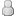• 11 Votes - 4.64 Average
• 1
• 2
• 3
• 4
• 5
01-11-2023, 01:50 PMPost: #391
 AxymeusJunior MemberPosts: 2 Joined: Dec 2022 Reputation: 0
So apparently the glitch I mentionned above is due to one cinematic rendering with an extra pixel in height, which breaks some shaders.

https://github.com/hrydgard/ppsspp/pull/12894

I have no doubt the team will update the built-in shaders to handle that properly, but I'd like to use a custom shader and I don't know how to update it... This is the current version, a port of a shader found in RetroArch.

Code:
```attribute vec4 a_position; attribute vec2 a_texcoord0; varying vec2 v_texcoord0; // vTexCoord varying vec2 v_texcoord1; // TextureSize uniform vec2 u_texelDelta; uniform vec4 u_setting; void main() {    v_texcoord0 = a_texcoord0;    v_texcoord1 = 1.0 / u_texelDelta;    gl_Position = a_position; }```

Code:
```#ifdef GL_ES precision mediump float; precision mediump int; #endif const float grid_strength = 0.04; const float target_gamma = 2.21; const float display_gamma = 2.2; const float saturation = 1.0; const float luminosity = 1.4; const float contrast = 1.0; const float rc = 0.98; const float gc = 0.795; const float bc = 0.98; const float rcg = 0.04; const float rcb = 0.01; const float gcr = 0.20; const float gcb = 0.01; const float bcr = -0.18; const float bcg = 0.165; uniform sampler2D sampler0; varying vec2 v_texcoord0;  // vTexCoord varying vec2 v_texcoord1;  // TextureSize float intsmear_func(float z) {    float z2 = z * z;    float z4 = z2 * z2;    float z8 = z4 * z4;    return z - 2.0 / 3.0 * z * z2 - 1.0 / 5.0 * z * z4          + 4.0 / 7.0 * z * z2 * z4 - 1.0 / 9.0 * z * z8          - 2.0 / 11.0 * z * z2 * z8 + 1.0 / 13.0 * z * z4 * z8; } float intsmear(float x, float dx) {    const float d = 1.5;    float zl = clamp((x - dx) / d, -1.0, 1.0);    float zh = clamp((x + dx) / d, -1.0, 1.0);    return d * (intsmear_func(zh) - intsmear_func(zl)) / (2.0 * dx); } void main() {      // LCD subpixel emulation    vec4 source_size = vec4(v_texcoord1, 1.0 / v_texcoord1);       vec2 texel_size = source_size.zw;    vec2 subtx_size = texel_size / vec2(3.0, 1.0);    vec2 range = source_size.xy / (vec2(1920, 1088) * source_size.xy);       float ltx = v_texcoord0.x - texel_size.x * 0.5;    float ttx = v_texcoord0.y + range.y;    float rtx = v_texcoord0.x + texel_size.x * 0.5;    float btx = v_texcoord0.y - range.y;       float subpix = mod(v_texcoord0.x / subtx_size.x + 1.5, 3.0);    float rsubpx = range.x / subtx_size.x;    vec4 lcol = vec4(          intsmear(subpix + 1.0, rsubpx),          intsmear(subpix, rsubpx),          intsmear(subpix - 1.0, rsubpx),          0.0);    vec4 rcol = vec4(          intsmear(subpix - 2.0, rsubpx),          intsmear(subpix - 3.0, rsubpx),          intsmear(subpix - 4.0, rsubpx),          0.0);             vec2 tl0 = (floor(vec2(ltx, ttx) / texel_size) + 0.5) * texel_size;    vec2 tr0 = (floor(vec2(rtx, ttx) / texel_size) + 0.5) * texel_size;    vec2 bl0 = (floor(vec2(ltx, btx) / texel_size) + 0.5) * texel_size;    vec2 br0 = (floor(vec2(rtx, btx) / texel_size) + 0.5) * texel_size;    vec4 tlc = pow(texture2D(sampler0, tl0), vec4(display_gamma)) * lcol;    vec4 trc = pow(texture2D(sampler0, tr0), vec4(display_gamma)) * rcol;    vec4 blc = pow(texture2D(sampler0, bl0), vec4(display_gamma)) * lcol;    vec4 brc = pow(texture2D(sampler0, br0), vec4(display_gamma)) * rcol;       vec2 border = floor((v_texcoord0.st / subtx_size) + 0.5);    vec2 bordet = clamp((border + vec2(0.0, +grid_strength)) * subtx_size,          vec2(ltx, btx), vec2(rtx, ttx));    vec2 bordeb = clamp((border + vec2(0.0, -grid_strength)) * subtx_size,          vec2(ltx, btx), vec2(rtx, ttx));       vec4 average_color;    float total_area = 2.0 * range.y;    average_color  = ((ttx - bordet.y) / total_area) * tlc;    average_color += ((bordeb.y - btx) / total_area) * brc;    average_color += ((bordeb.y - btx) / total_area) * blc;    average_color += ((ttx - bordet.y) / total_area) * trc;       vec4 current = pow(average_color, vec4(1.0 / display_gamma));       // Color regrading to simulate the LCD display    vec4 screen = pow(current, vec4(target_gamma));    vec4 avglum = vec4(0.5);    screen = mix(screen, avglum, (1.0 - contrast));       mat4 color = mat4(          rc, rcg, rcb, 0.0,          gcr, gc, gcb, 0.0,          bcr, bcg, bc, 0.0,          0.0, 0.0, 0.0, 0.0);             float rad = (1.0 - saturation) * 0.3086;    float gad = (1.0 - saturation) * 0.6094;    float bad = (1.0 - saturation) * 0.0820;    mat4 adjust = mat4(          rad + saturation, rad, rad, 1.0,          gad, gad + saturation, gad, 1.0,          bad, bad, bad + saturation, 1.0,          0.0, 0.0, 0.0, 1.0);             color = color * adjust;    screen = clamp(screen * luminosity, 0.0, 1.0);    screen = color * screen;       gl_FragColor = pow(screen, vec4(1.0 / display_gamma)); }```

Maybe someone can help me with that? The shader also assumes an output resolution of 1920x1088 (4x the PSP resolution) but it's hard-coded. It would be better to use the display size.

I'm not big on programming and just managed to make this one work lol. Any help is appreciated thank you.
 « Next Oldest | Next Newest »